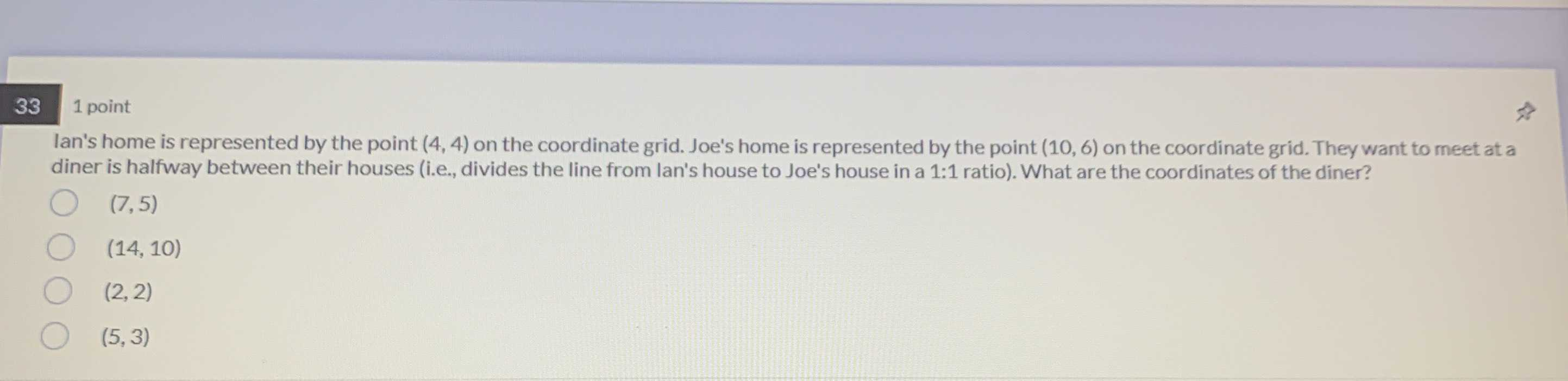### Still have math questions?

Algebra
Questionlan's home is represented by the point $$( 4,4 )$$ on the coordinate grid. Joe's home is represented by the point $$( 10,6 )$$ on the coordinate grid. They want to meet at a diner is halfway between their houses (i.e., divides the line from lan's house to Joe's house in a $$1 : 1$$ ratio). What are the coordinates of the diner?

$$( 7,5 )$$

$$( 14,10 )$$

$$( 2,2 )$$

$$( 5,3 )$$

x= $$\frac{10+ 4}{2}= 7$$
y=$$\frac{6+ 4}{2}$$=5